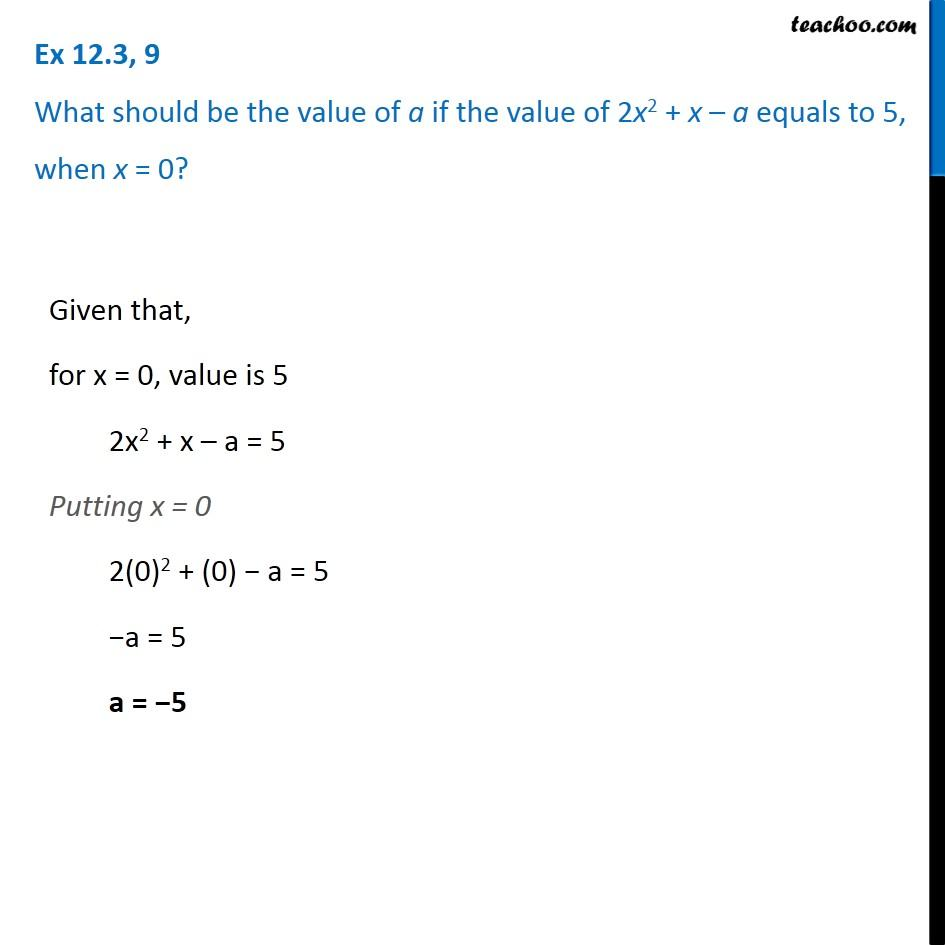Ex 12.3

Chapter 12 Class 7 Algebraic Expressions
Serial order wise### Transcript

Ex 12.3, 9 What should be the value of a if the value of 2x2 + x – a equals to 5, when x = 0? Given that, for x = 0, value is 5 2x2 + x – a = 5 Putting x = 0 2(0)2 + (0) − a = 5 −a = 5 a = −5